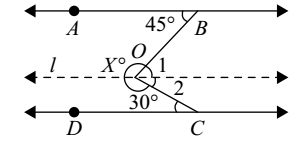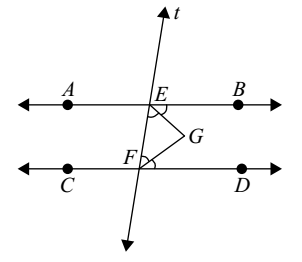## Plane Geometry

#### Plane Geometry

1. Two angles are supplementary and the ratio of the angels is 1:4. what is the value of smaller angle?
1. 36 degree
2. 45 degree
3. 35 degree
4. 72 degree

1. Since the angles are supplementary therefore the sum of their angles will be 180 degree.

##### Correct Option: A

As we know that the angles are supplementary so sum of angles will be 180 degree.
Let us assume that the ratio factor is r.
According to question,
Angles are supplementary and have a ratio of 1:4.
r + 4r = 180
⇒ 5r = 180
⇒ r = 180/5
⇒ r = 36

1. The complement of 30°20′ is:
1. 69°40′
2. 59°40′
3. 35°80′
4. 159°40′
5. None of these

1. complement of 30°20′ = 90° – ( 30°20′ ) = 90° – ( 30° + 20′ )
= (89° – 30°) + (1° – 20′)

##### Correct Option: B

complement of 30°20′ = 90° – ( 30°20′ ) = 90° – ( 30° + 20′ )
= (89° – 30°) + (1° – 20′)
= 59° + 60′ – 20′ [ ∴ 1° = 60°′]
= 59° + 40′ = 59°40′.

1. In the given, AB || CD. Then X is equal to:1. 93°
2. 103°
3. 83°
4. 97°
5. None of these

1. Through O, draw a line l parallel to both AB and CD. Then
∠1 = 45° (alt. ∠S)
and ∠2 = 30° (alt. ∠S)
∴ ∠BOC = ∠1 + ∠2 = 45° + 30° = 75°

##### Correct Option: E

Through O, draw a line l parallel to both AB and CD. Then
∠1 = 45° (alt. ∠S)
and ∠2 = 30° (alt. ∠S)
∴ ∠BOC = ∠1 + ∠2 = 45° + 30° = 75°
So, X = 360° – ∠BOC = 360° – 75° = 285°
Hence X = 285°.

1. An angle is equal to one-third of its supplement. Its measure is equal to :
1. 40°
2. 50°
3. 45°
4. 55°
5. None of these

1. Let the measured of the required angle be P degree.
Then, its supplement = 180 – P
Now use the formula,

 Angle = 1 ( its supplement ) 3

##### Correct Option: C

Let the measured of the required angle be P degree.
Then, its supplement = 180 – P
Now use the formula,

 Angle = 1 ( its supplement ) 3

 P = ( 180 - P ) 3

3P + P 180°
⇒ P = 45°

1. In the adjoining figure, AB || CD, t is the traversal, EG and FG are the bisectors of ∠BEE and ∠DFE respectively, then ∠EGF is equal to :1. 90°
2. 75°
3. 80°
4. 110°
5. None of these

1. AB || CD and t transversal intersects them at E and F
∠BEF + ∠EFD = 180° (co-interior angles)

 ⇒ 1 ∠BEF + 1 ∠EFD = 180/2 2 2

⇒ ∠FEG + ∠EFG = 90°
In Δ GEF
∠EGF + ∠FEG + ∠EFG = 180°

##### Correct Option: A

AB || CD and t transversal intersects them at E and F
∠BEF + ∠EFD = 180° (co-interior angles)

 ⇒ 1 ∠BEF + 1 ∠EFD = 180/2 2 2

⇒ ∠FEG + ∠EFG = 90°
In Δ GEF
∠EGF + ∠FEG + ∠EFG = 180°
∴ ∠EGF + 90° = 180°
∴ ∠EGF = 90°.
The above result can be restated as :
If two parallel lines are cut by a traversal, then the bisectors of the interior angles on the same side of the traversal intersect each other at right angles.